# NCERT Exemplar Class 10 Science Solutions for Chapter 1 - Chemical Reactions And Equations

NCERT Exemplar Class 10 Science Chapter 1 Chemical Reactions and Equations will help you in getting an understanding of all the fundamentals of chemical reactions as well as their symbolic representation in terms of equations. Here students will recognize chemical changes, write equations, classify chemical reactions and basically list a few examples of chemical reactions.

Thus, to help students get a clear idea about the chapter and the topics, we are offering free NCERT exemplar for class 10 Science chapter 1 – chemical reactions and equations here. The exemplar is prepared by experts and is designed to help students get the right answers for questions, as well as, have a thorough revision of the entire chapter. The exemplar act as a very resourceful study tool and will help students to perform excellently in the exams.

### Download PDF of NCERT exemplar for Class 10 Science Chapter 1 – Chemical Reactions and Equations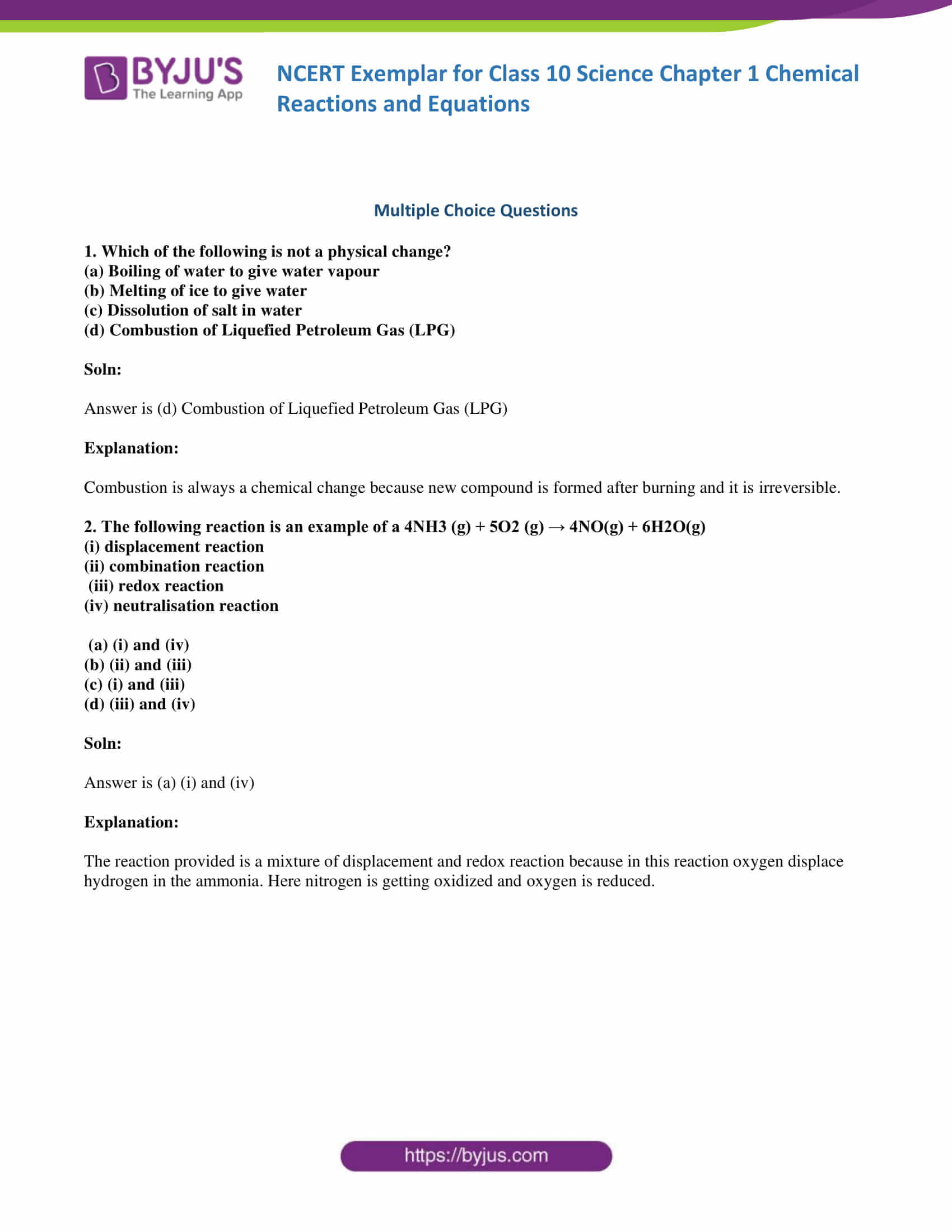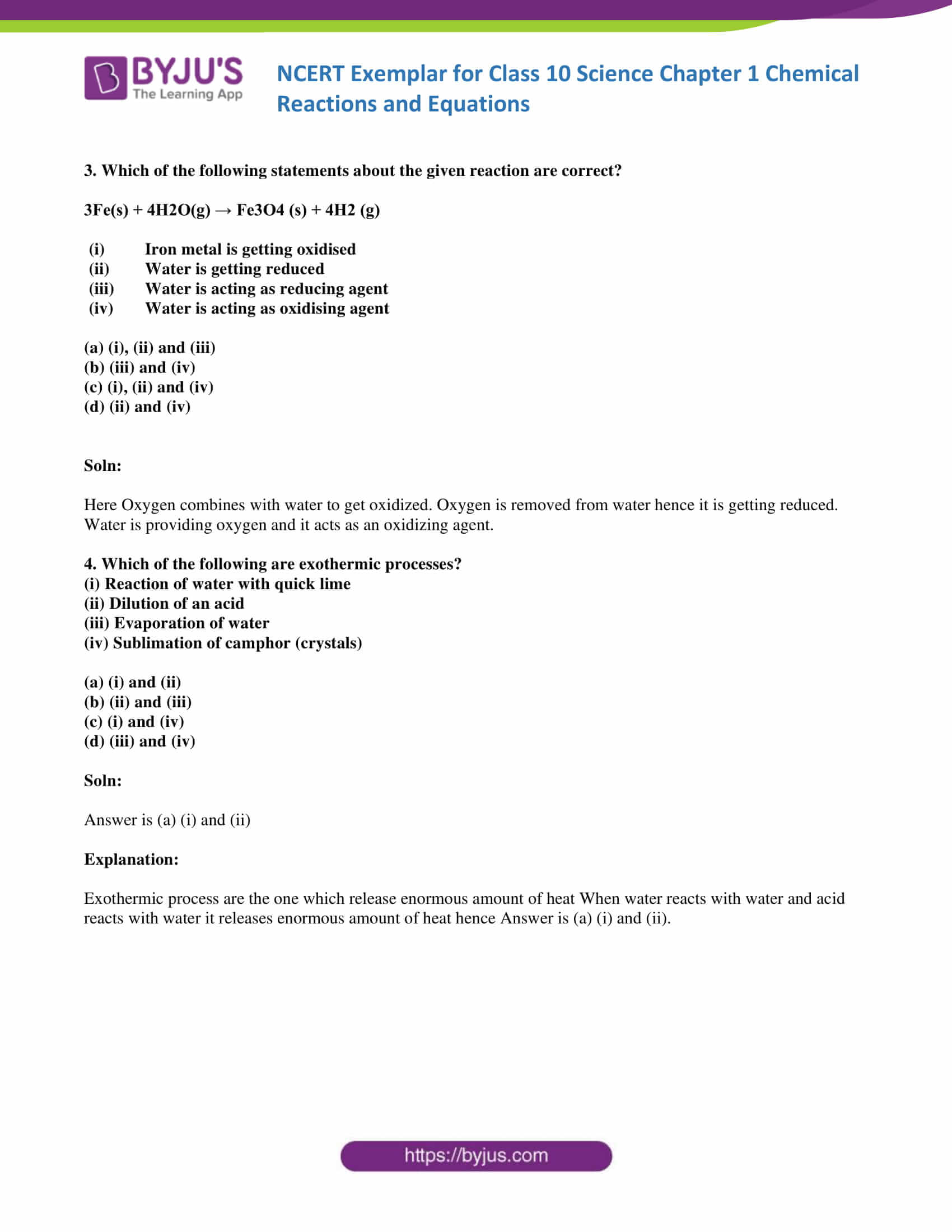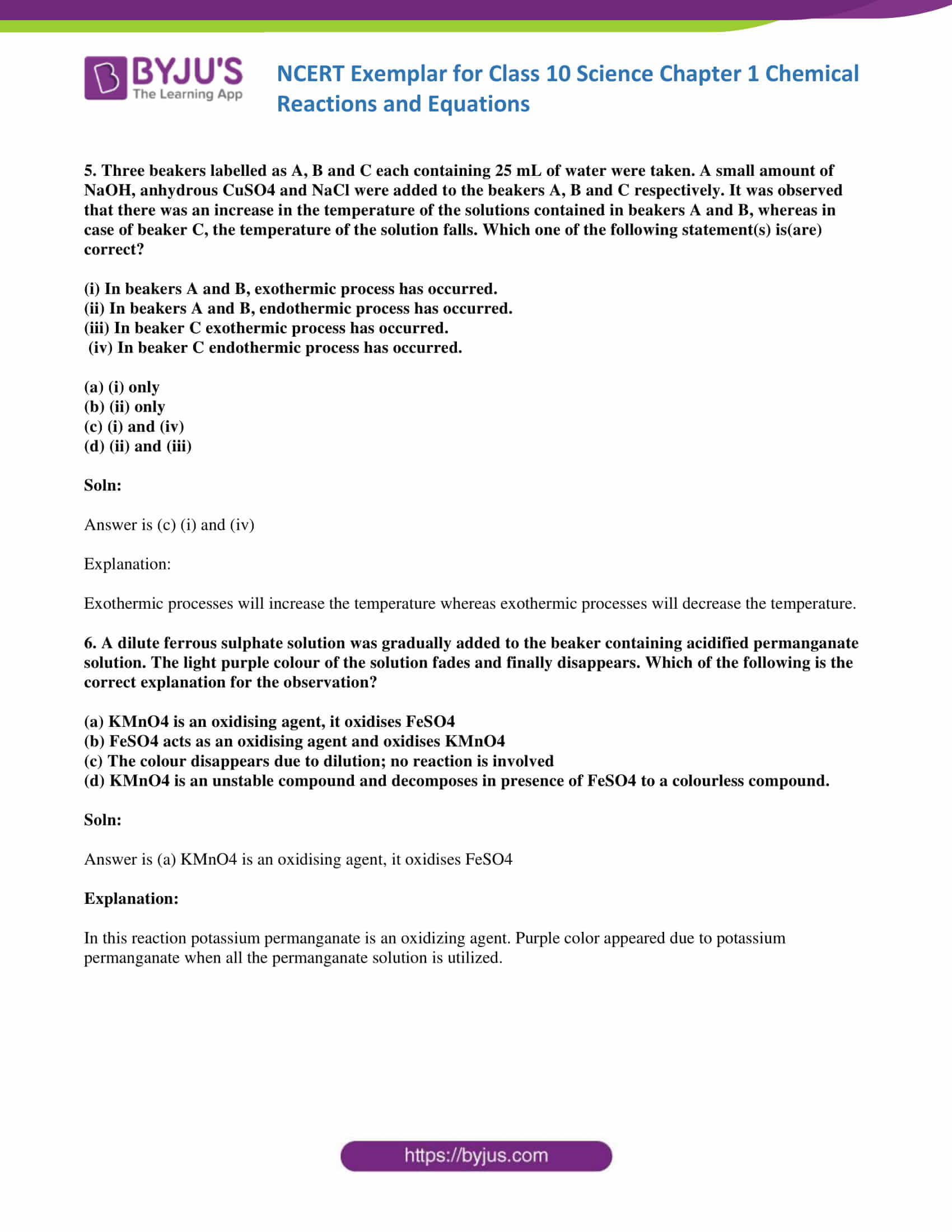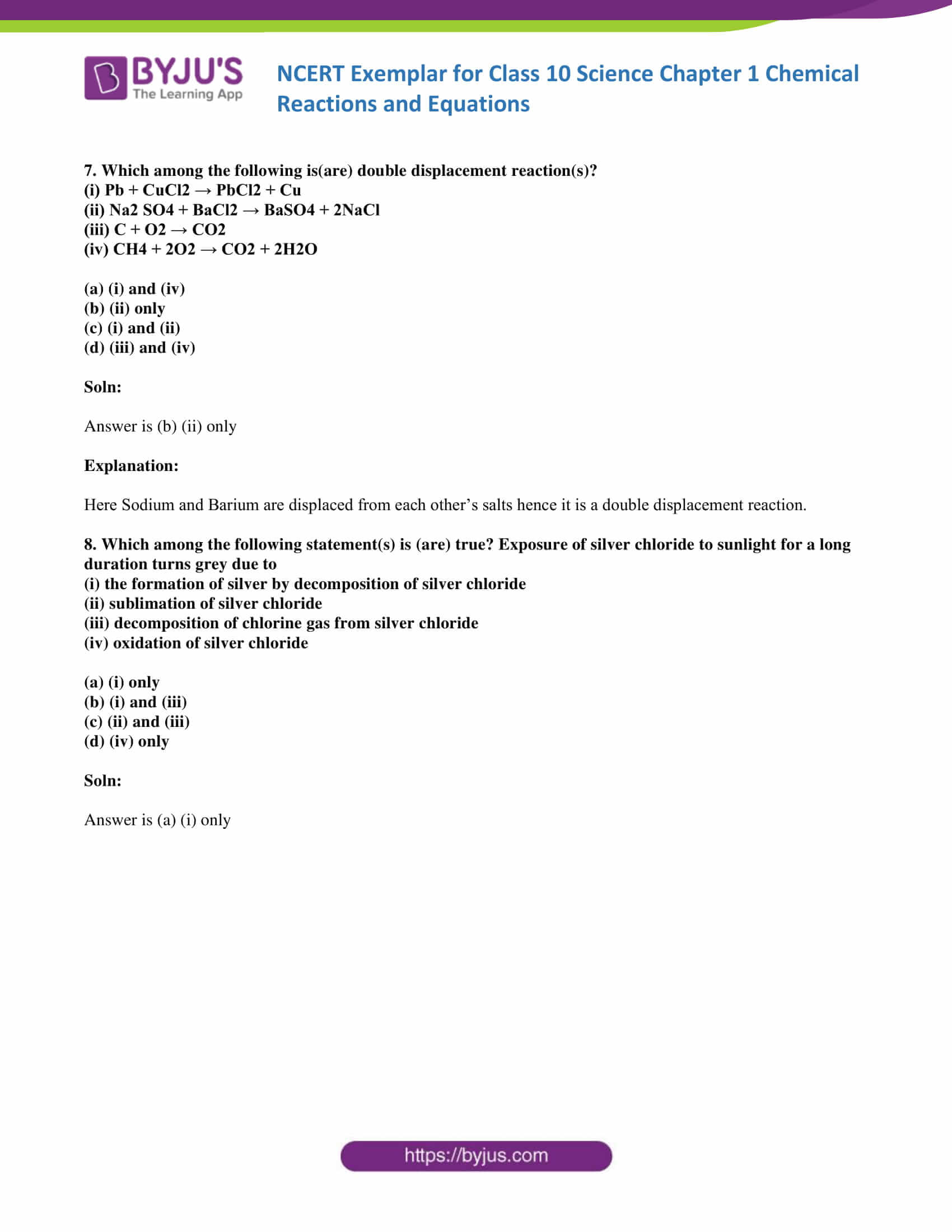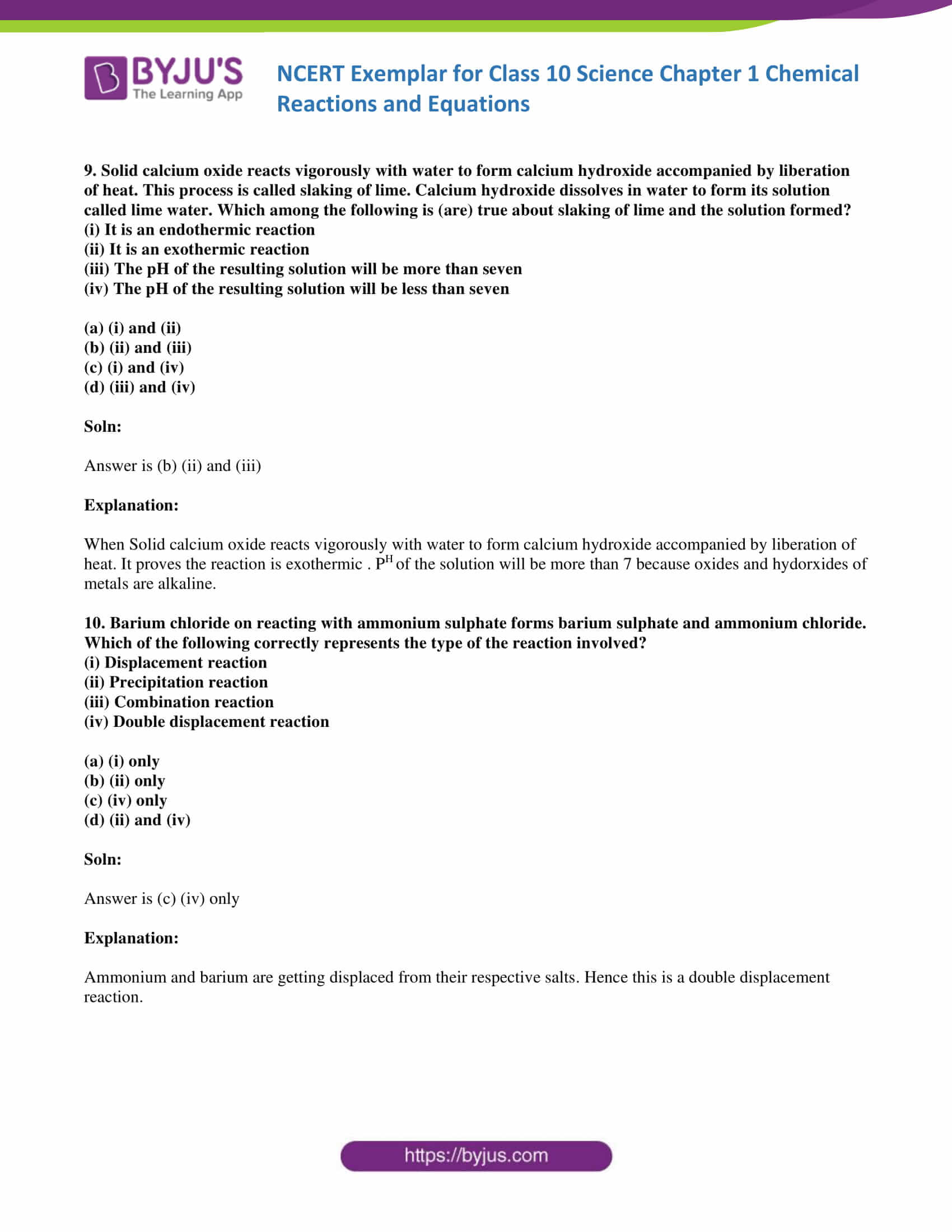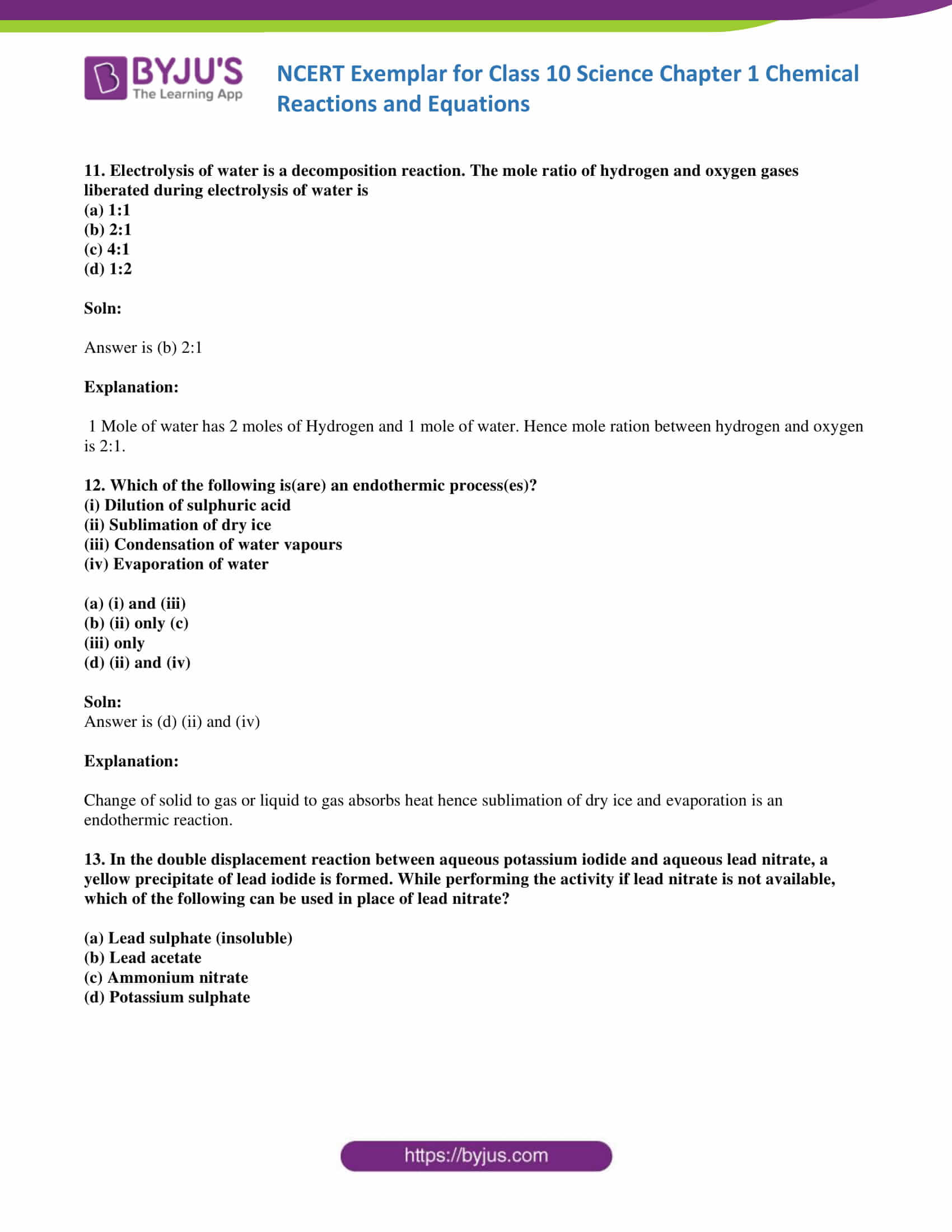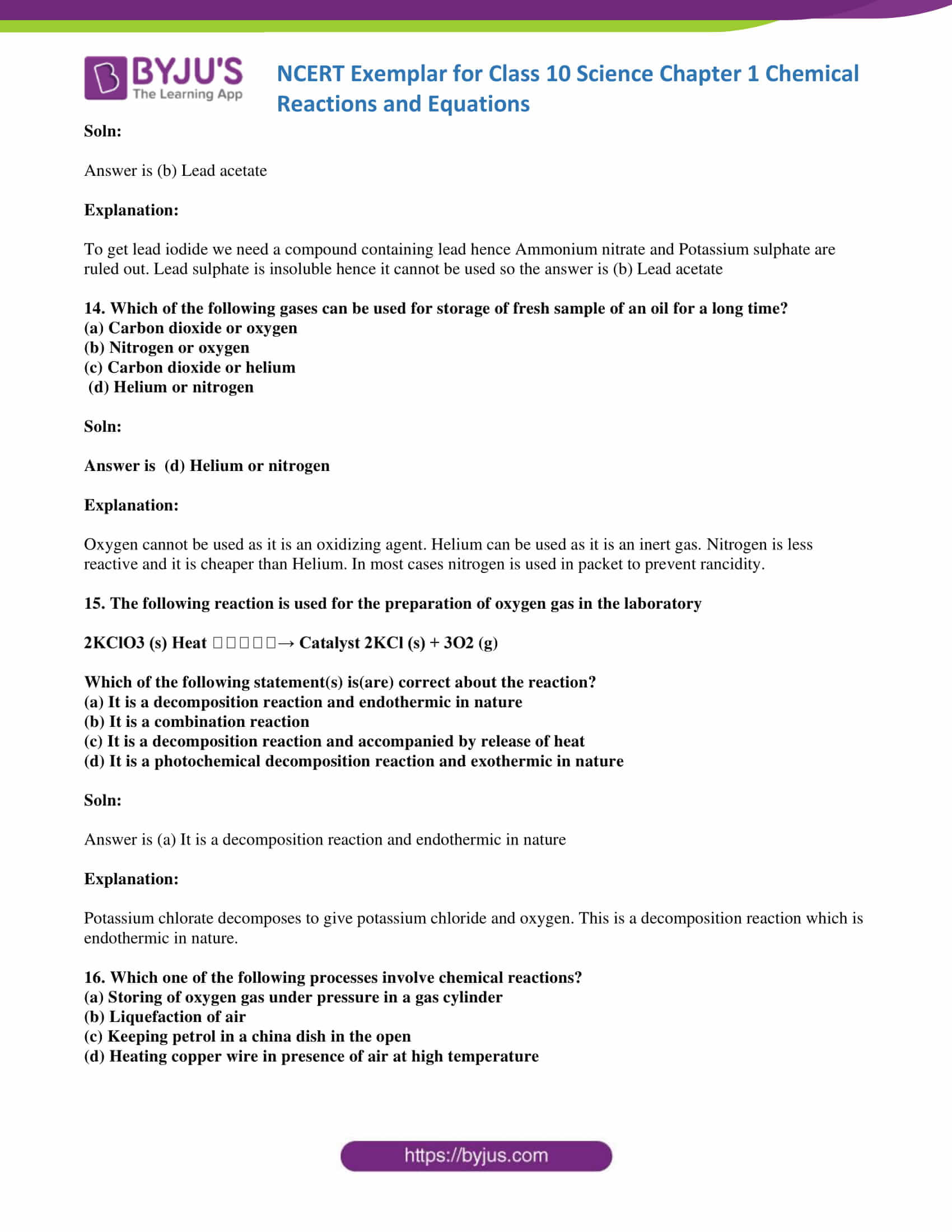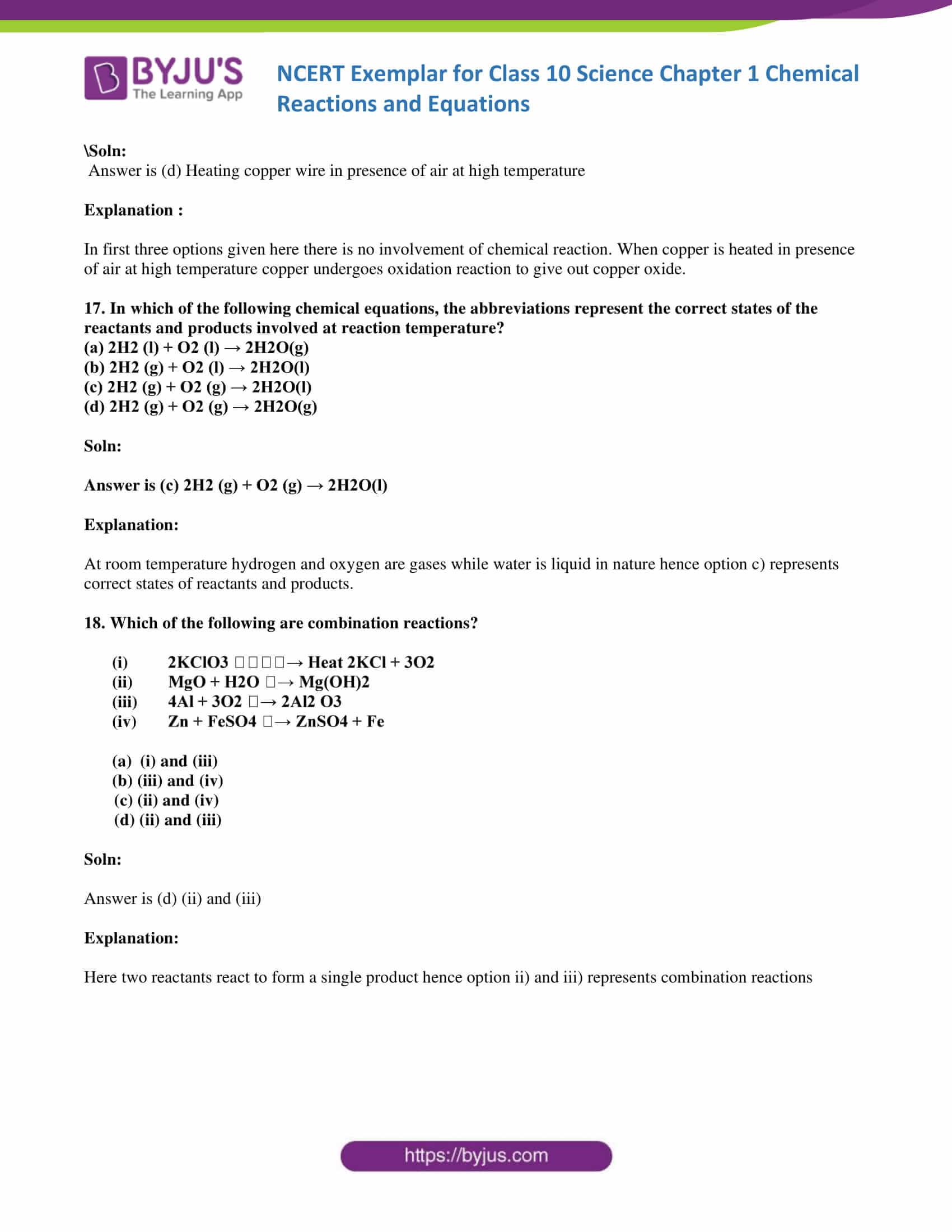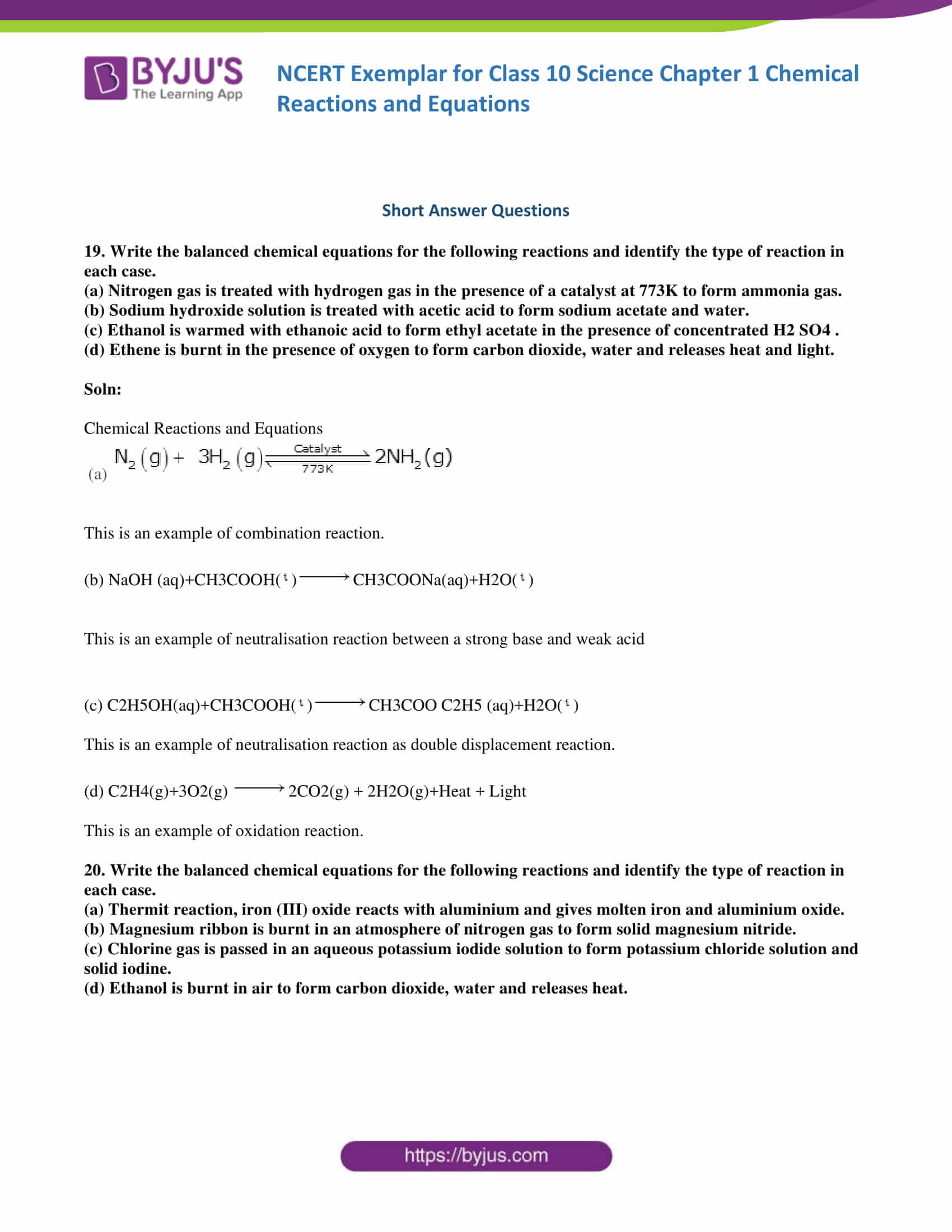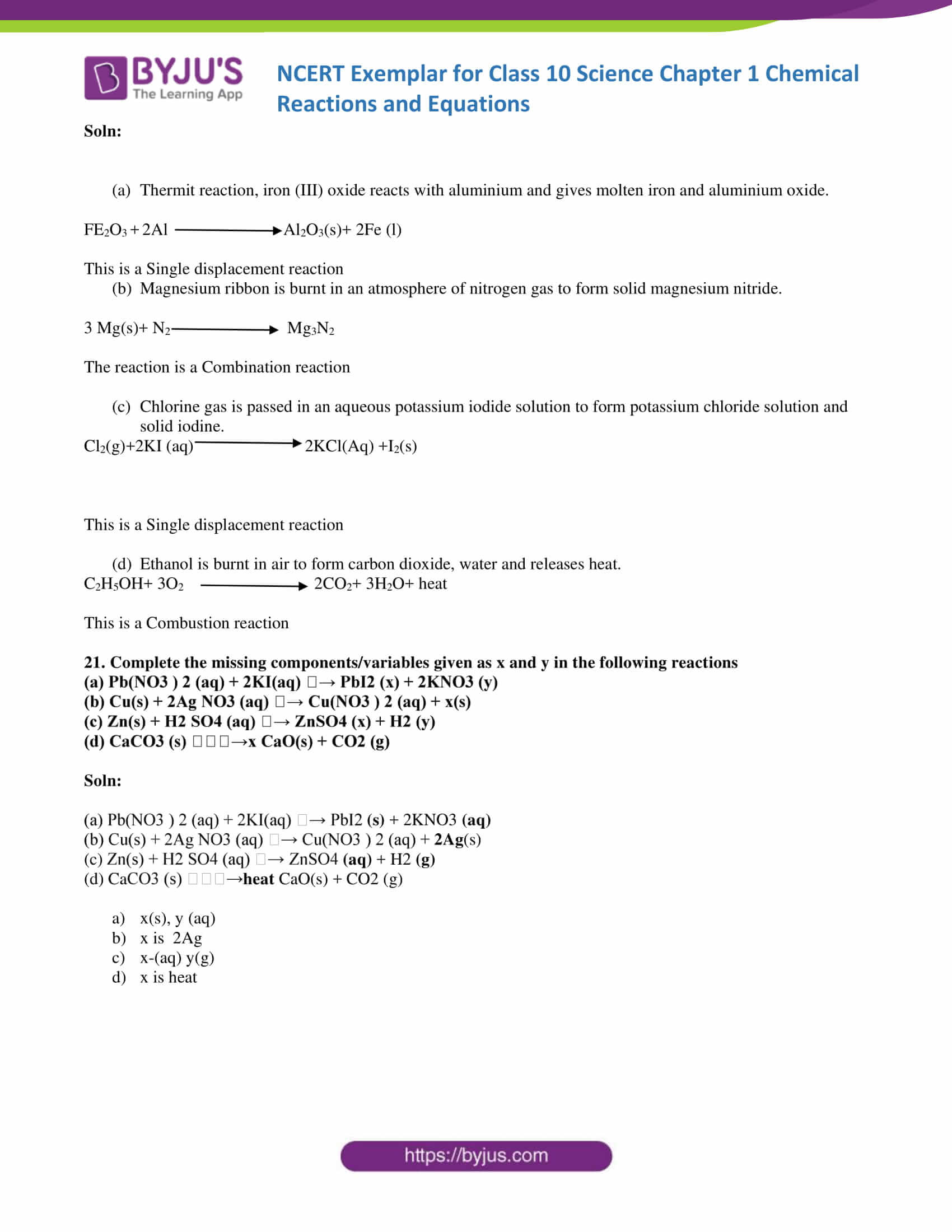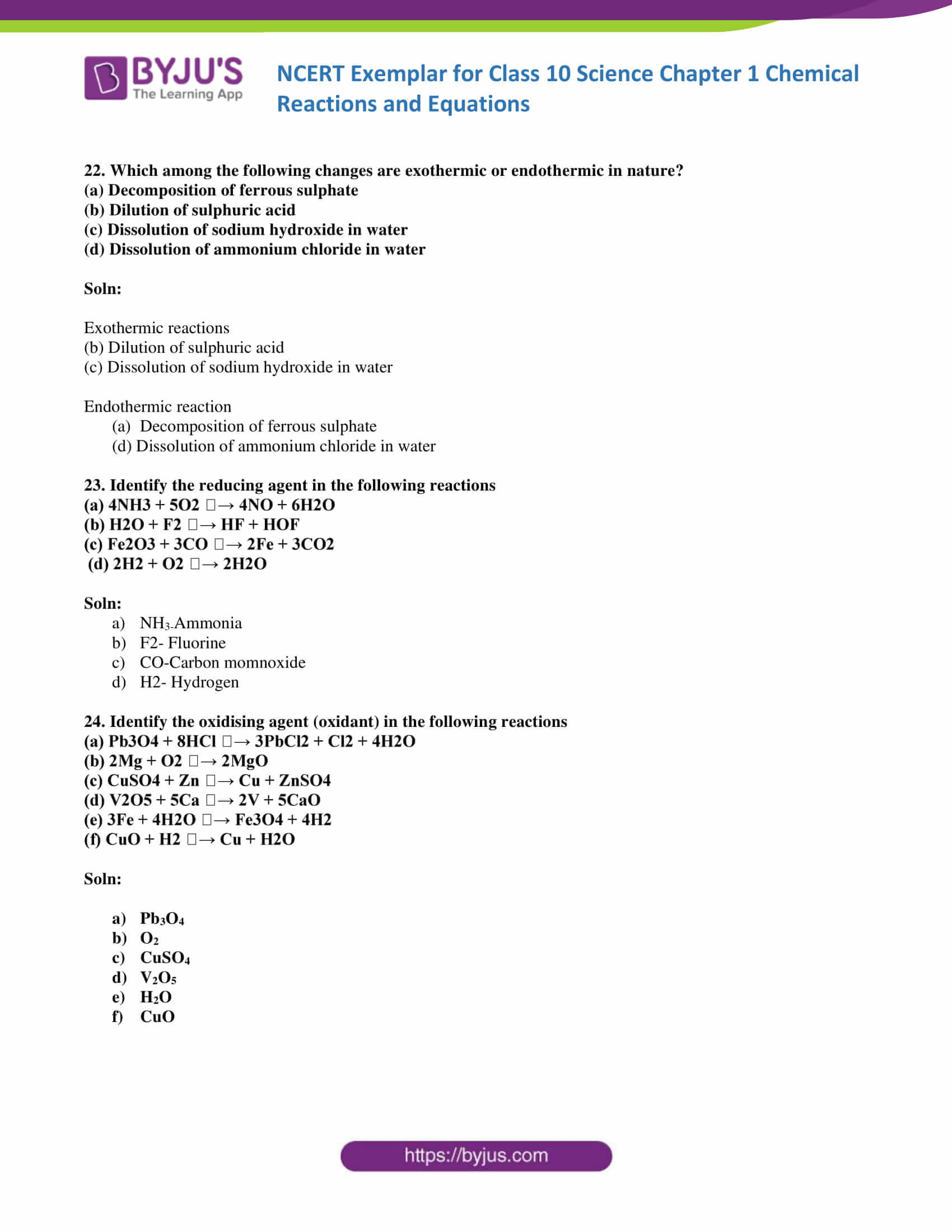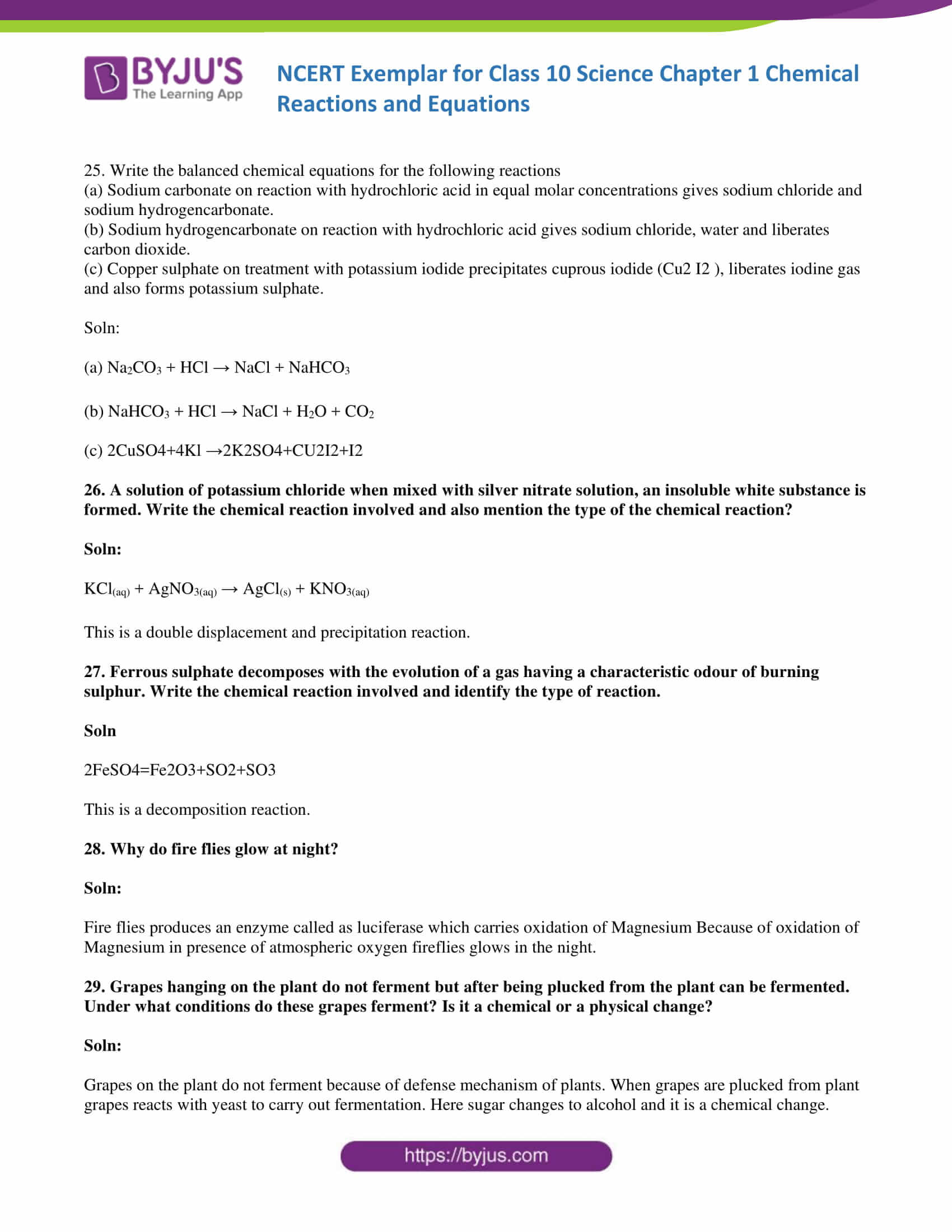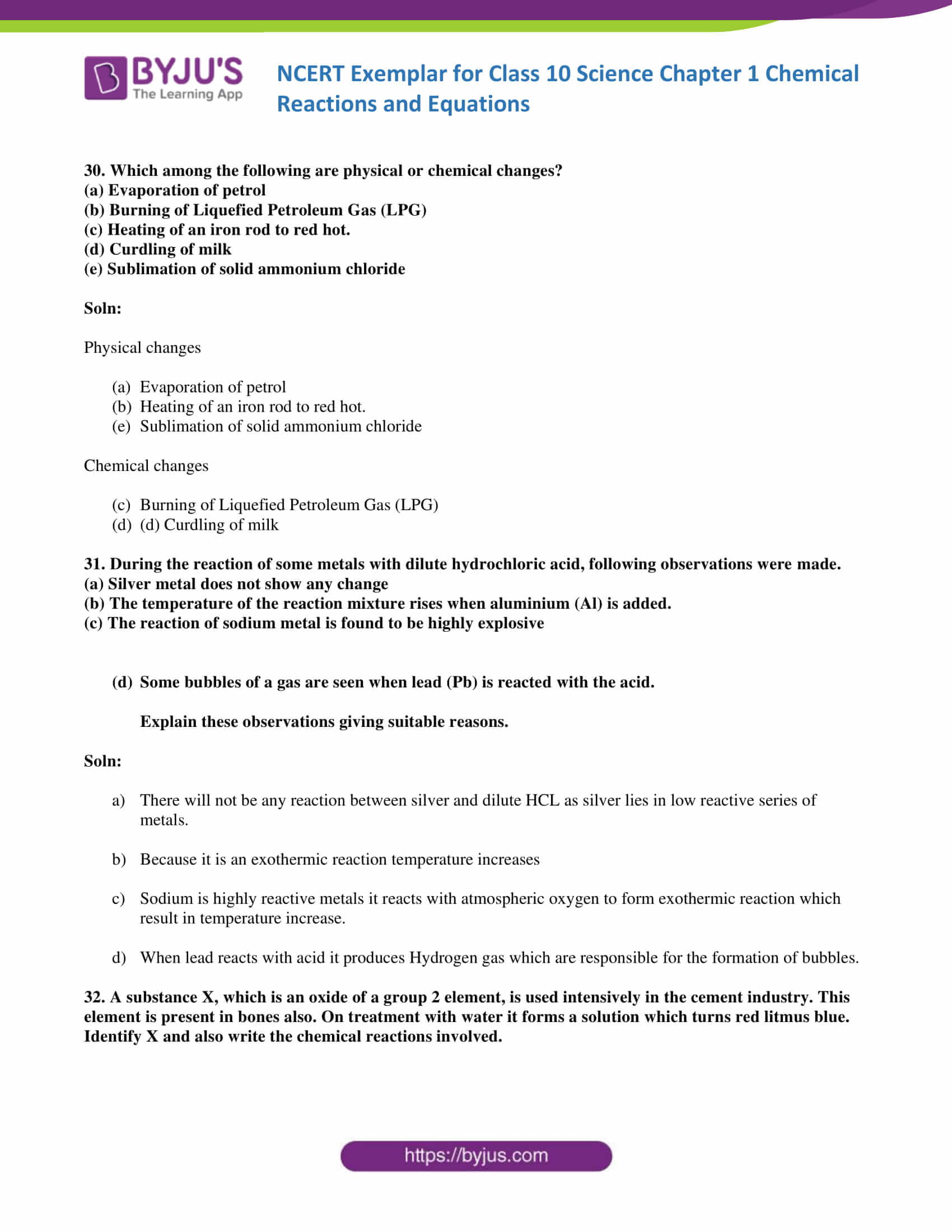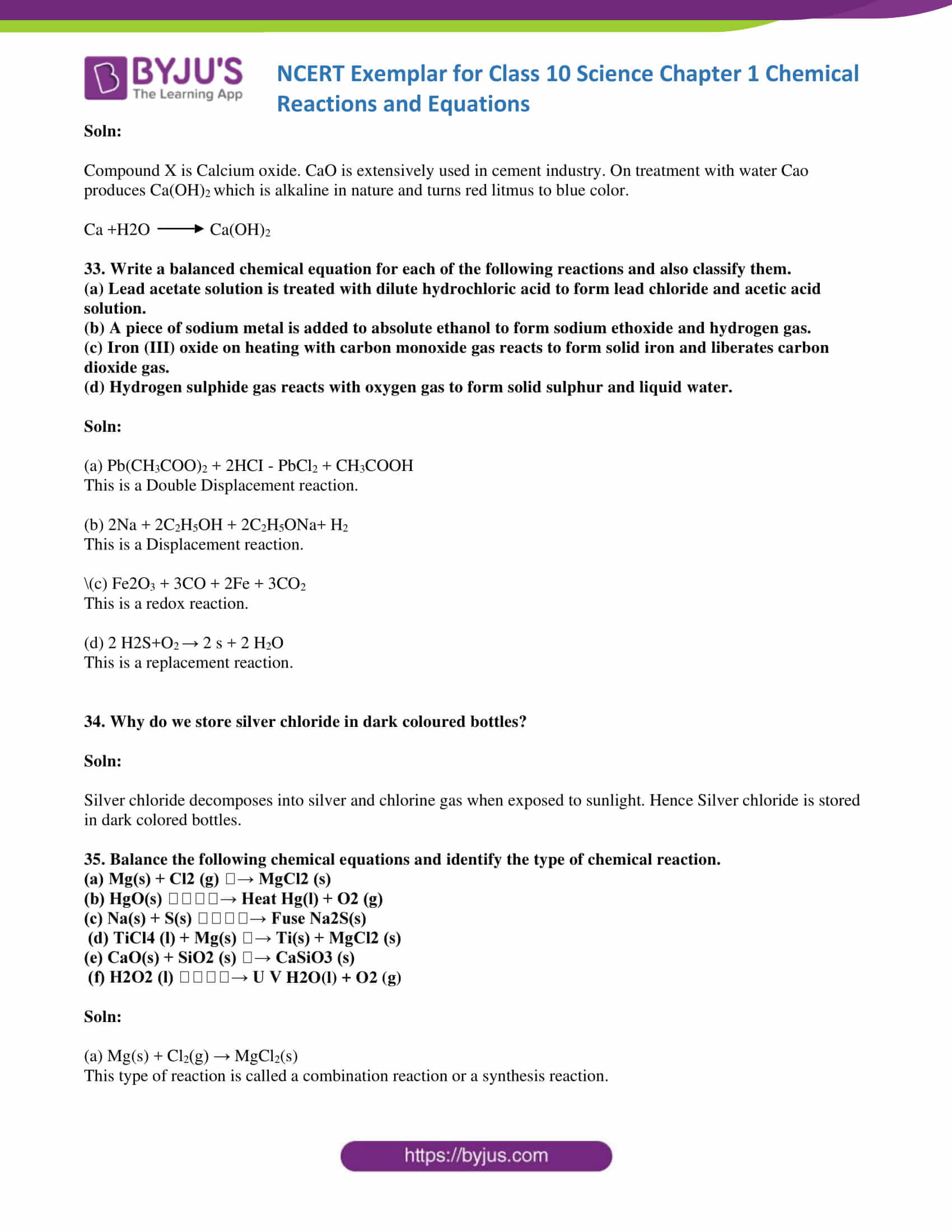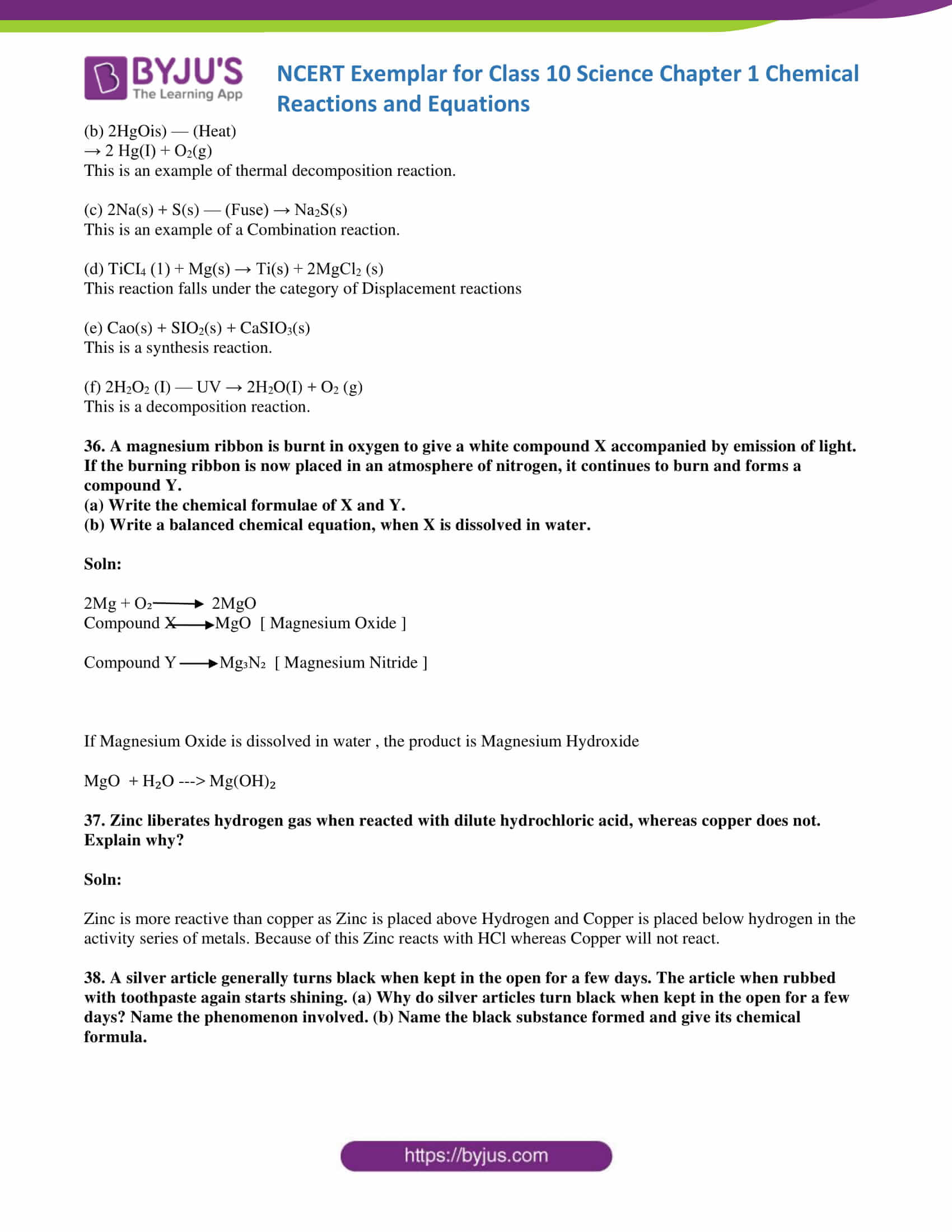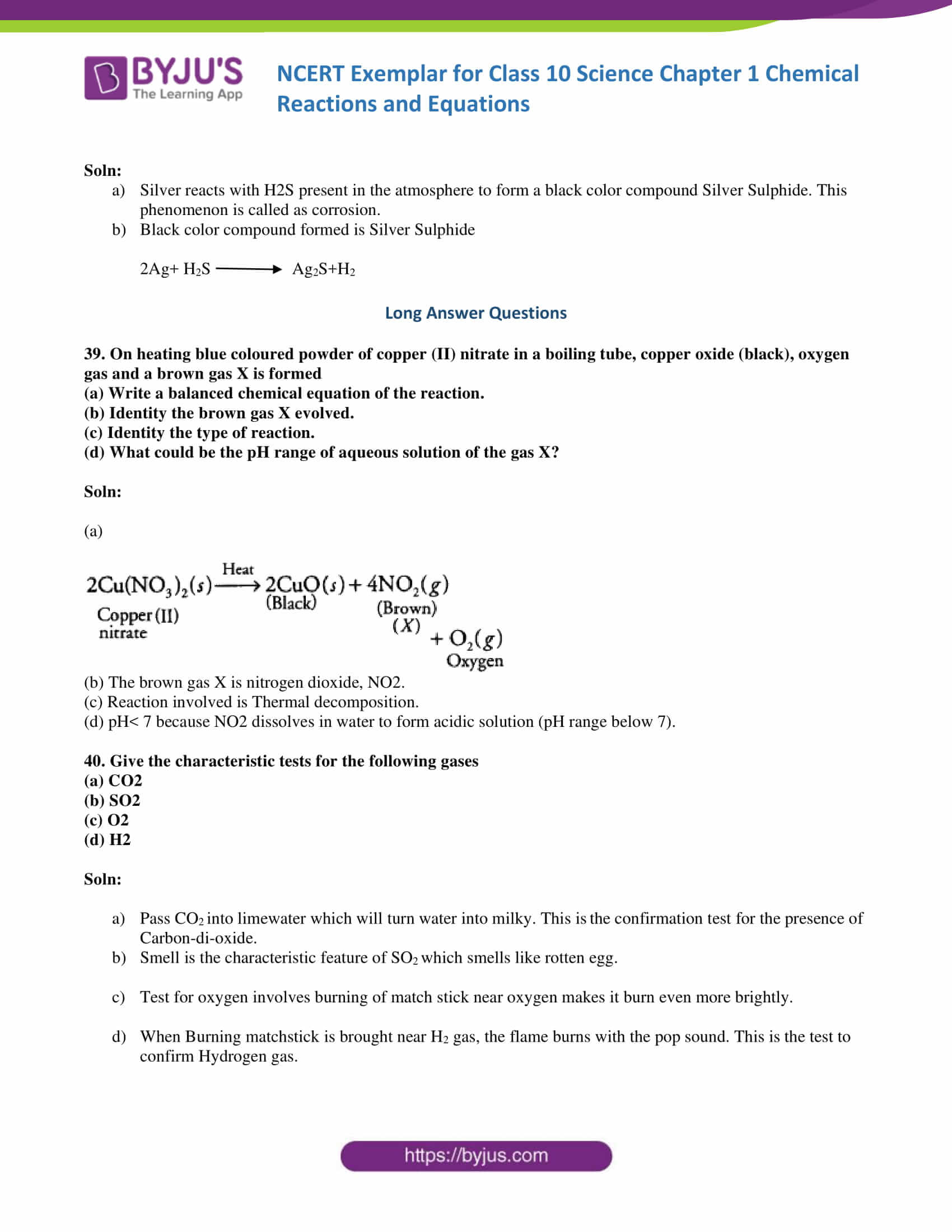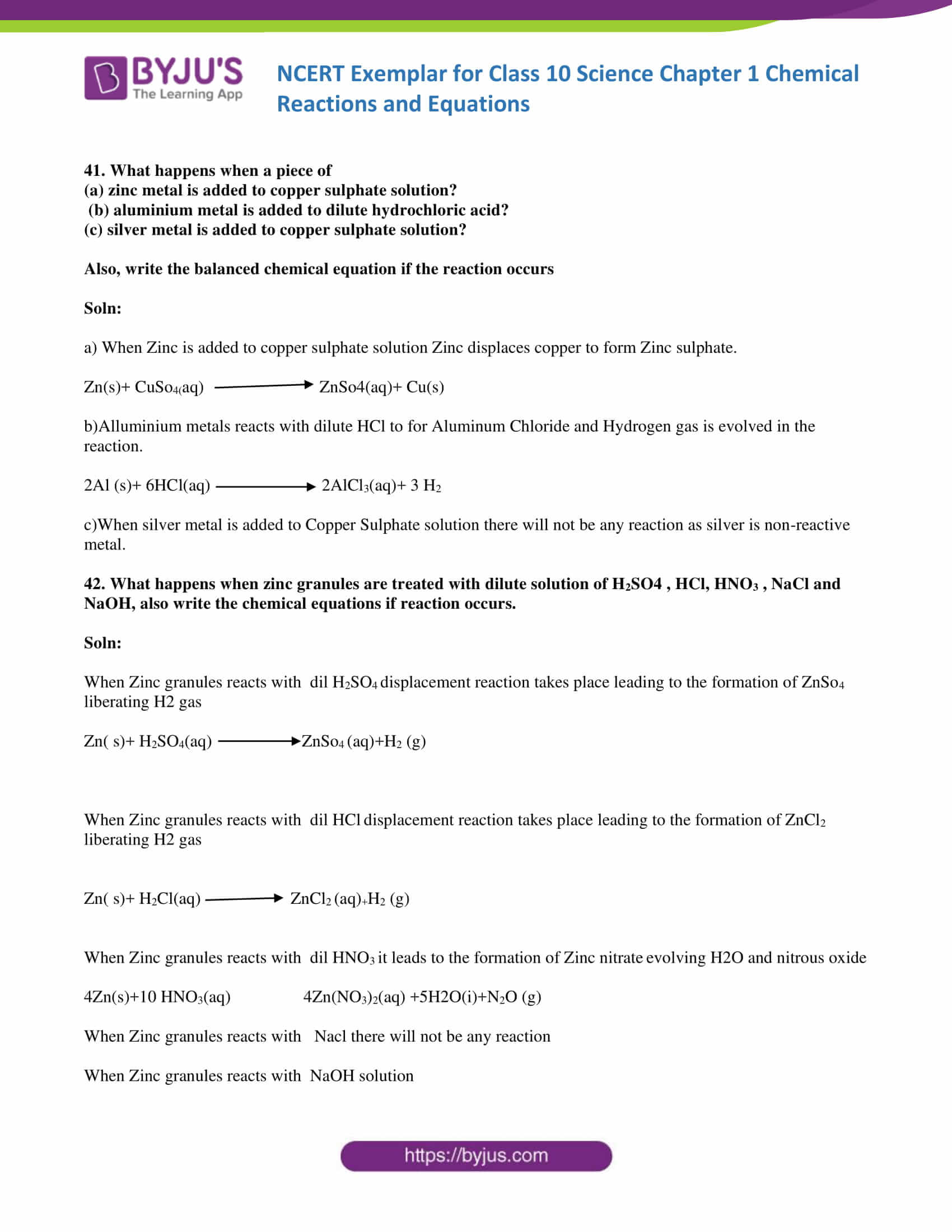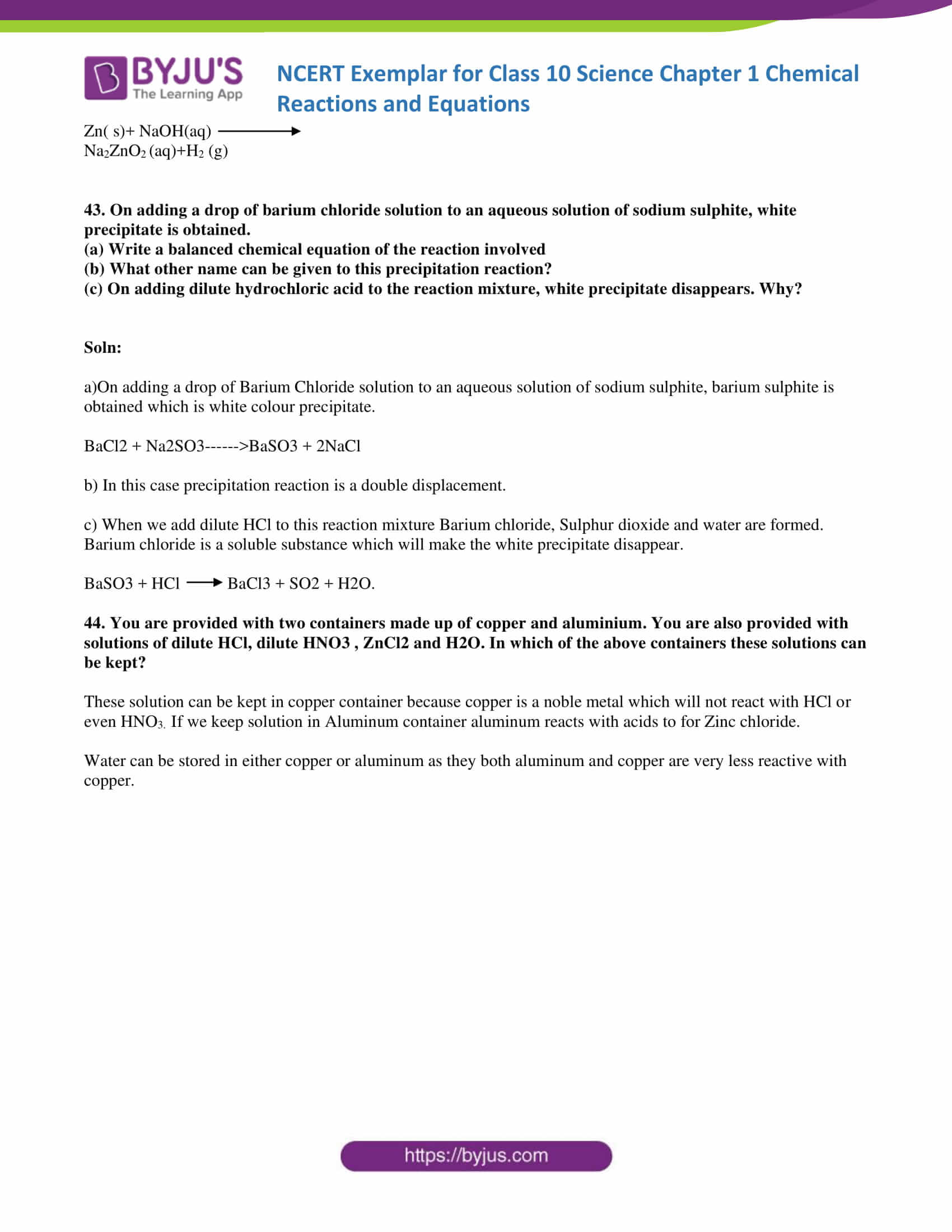### Multiple Choice Questions

1. Which of the following is not a physical change?

(a) Boiling of water to give water vapour

(b) Melting of ice to give water

(c) Dissolution of salt in water

(d) Combustion of Liquefied Petroleum Gas (LPG)

Soln:

Answer is (d) Combustion of Liquefied Petroleum Gas (LPG)

Explanation:

Combustion is always a chemical change because new compound is formed after burning and it is irreversible.

2. The following reaction is an example of a 4NH3 (g) + 5O2 (g) → 4NO(g) + 6H2O(g)

(i) displacement reaction

(ii) combination reaction

(iii) redox reaction

(iv) neutralisation reaction

(a) (i) and (iv)

(b) (ii) and (iii)

(c) (i) and (iii)

(d) (iii) and (iv)

Soln:

Answer is (a) (i) and (iv)

Explanation:

The reaction provided is a mixture of displacement and redox reaction because in this reaction oxygen displace hydrogen in the ammonia. Here nitrogen is getting oxidized and oxygen is reduced.

3. Which of the following statements about the given reaction are correct?

3Fe(s) + 4H2O(g) → Fe3O4 (s) + 4H2 (g)

1. Iron metal is getting oxidised
2. Water is getting reduced
3. Water is acting as reducing agent
4. Water is acting as oxidising agent

(a) (i), (ii) and (iii)

(b) (iii) and (iv)

(c) (i), (ii) and (iv)

(d) (ii) and (iv)

Soln:

Here Oxygen combines with water to get oxidized. Oxygen is removed from water hence it is getting reduced. Water is providing oxygen and it acts as an oxidizing agent.

4. Which of the following are exothermic processes?

(i) Reaction of water with quick lime

(ii) Dilution of an acid

(iii) Evaporation of water

(iv) Sublimation of camphor (crystals)

(a) (i) and (ii)

(b) (ii) and (iii)

(c) (i) and (iv)

(d) (iii) and (iv)

Soln:

Answer is (a) (i) and (ii)

Explanation:

Exothermic process are the one which release enormous amount of heat When water reacts with water and acid reacts with water it releases enormous amount of heat hence Answer is (a) (i) and (ii).

5. Three beakers labelled as A, B and C each containing 25 mL of water were taken. A small amount of NaOH, anhydrous CuSO4 and NaCl were added to the beakers A, B and C respectively. It was observed that there was an increase in the temperature of the solutions contained in beakers A and B, whereas in case of beaker C, the temperature of the solution falls. Which one of the following statement(s) is(are) correct?

(i) In beakers A and B, exothermic process has occurred.

(ii) In beakers A and B, endothermic process has occurred.

(iii) In beaker C exothermic process has occurred.

(iv) In beaker C endothermic process has occurred.

(a) (i) only

(b) (ii) only

(c) (i) and (iv)

(d) (ii) and (iii)

Soln:

Answer is (c) (i) and (iv)

Explanation:

Exothermic processes will increase the temperature whereas exothermic processes will decrease the temperature.

6. A dilute ferrous sulphate solution was gradually added to the beaker containing acidified permanganate solution. The light purple colour of the solution fades and finally disappears. Which of the following is the correct explanation for the observation?

(a) KMnO4 is an oxidising agent, it oxidises FeSO4

(b) FeSO4 acts as an oxidising agent and oxidises KMnO4

(c) The colour disappears due to dilution; no reaction is involved

(d) KMnO4 is an unstable compound and decomposes in presence of FeSO4 to a colourless compound.

Soln:

Answer is (a) KMnO4 is an oxidising agent, it oxidises FeSO4

Explanation:

In this reaction potassium permanganate is an oxidizing agent. Purple color appeared due to potassium permanganate when all the permanganate solution is utilized.

7. Which among the following is(are) double displacement reaction(s)?

(i) Pb + CuCl2 → PbCl2 + Cu

(ii) Na2 SO4 + BaCl2 → BaSO4 + 2NaCl

(iii) C + O2 → CO2

(iv) CH4 + 2O2 → CO2 + 2H2O

(a) (i) and (iv)

(b) (ii) only

(c) (i) and (ii)

(d) (iii) and (iv)

Soln:

Explanation:

Here Sodium and Barium are displaced from each other’s salts hence it is a double displacement reaction.

8. Which among the following statement(s) is (are) true? Exposure of silver chloride to sunlight for a long duration turns grey due to

(i) the formation of silver by decomposition of silver chloride

(ii) sublimation of silver chloride

(iii) decomposition of chlorine gas from silver chloride

(iv) oxidation of silver chloride

(a) (i) only

(b) (i) and (iii)

(c) (ii) and (iii)

(d) (iv) only

Soln:

9. Solid calcium oxide reacts vigorously with water to form calcium hydroxide accompanied by liberation of heat. This process is called slaking of lime. Calcium hydroxide dissolves in water to form its solution called lime water. Which among the following is (are) true about slaking of lime and the solution formed?

(i) It is an endothermic reaction

(ii) It is an exothermic reaction

(iii) The pH of the resulting solution will be more than seven

(iv) The pH of the resulting solution will be less than seven

(a) (i) and (ii)

(b) (ii) and (iii)

(c) (i) and (iv)

(d) (iii) and (iv)

Soln:

Answer is (b) (ii) and (iii)

Explanation:

When Solid calcium oxide reacts vigorously with water to form calcium hydroxide accompanied by liberation of heat. It proves the reaction is exothermic . PH of the solution will be more than 7 because oxides and hydorxides of metals are alkaline.

10. Barium chloride on reacting with ammonium sulphate forms barium sulphate and ammonium chloride. Which of the following correctly represents the type of the reaction involved?

(i) Displacement reaction

(ii) Precipitation reaction

(iii) Combination reaction

(iv) Double displacement reaction

(a) (i) only

(b) (ii) only

(c) (iv) only

(d) (ii) and (iv)

Soln:

Explanation:

Ammonium and barium are getting displaced from their respective salts. Hence this is a double displacement reaction.

11. Electrolysis of water is a decomposition reaction. The mole ratio of hydrogen and oxygen gases liberated during electrolysis of water is

(a) 1:1

(b) 2:1

(c) 4:1

(d) 1:2

Soln:

Explanation:

1 Mole of water has 2 moles of Hydrogen and 1 mole of water. Hence mole ration between hydrogen and oxygen is 2:1.

12. Which of the following is(are) an endothermic process(es)?

(i) Dilution of sulphuric acid

(ii) Sublimation of dry ice

(iii) Condensation of water vapours

(iv) Evaporation of water

(a) (i) and (iii)

(b) (ii) only (c)

(iii) only

(d) (ii) and (iv)

Soln:

Answer is (d) (ii) and (iv)

Explanation:

Change of solid to gas or liquid to gas absorbs heat hence sublimation of dry ice and evaporation is an endothermic reaction.

13. In the double displacement reaction between aqueous potassium iodide and aqueous lead nitrate, a yellow precipitate of lead iodide is formed. While performing the activity if lead nitrate is not available, which of the following can be used in place of lead nitrate?

(c) Ammonium nitrate

(d) Potassium sulphate

Soln:

Explanation:

To get lead iodide we need a compound containing lead hence Ammonium nitrate and Potassium sulphate are ruled out. Lead sulphate is insoluble hence it cannot be used so the answer is (b) Lead acetate

14. Which of the following gases can be used for storage of fresh sample of an oil for a long time?

(a) Carbon dioxide or oxygen

(b) Nitrogen or oxygen

(c) Carbon dioxide or helium

(d) Helium or nitrogen

Soln:

Answer is (d) Helium or nitrogen

Explanation:

Oxygen cannot be used as it is an oxidizing agent. Helium can be used as it is an inert gas. Nitrogen is less reactive and it is cheaper than Helium. In most cases nitrogen is used in packet to prevent rancidity.

15. The following reaction is used for the preparation of oxygen gas in the laboratory

2KClO3 (s) Heat → Catalyst 2KCl (s) + 3O2 (g)

Which of the following statement(s) is(are) correct about the reaction?

(a) It is a decomposition reaction and endothermic in nature

(b) It is a combination reaction

(c) It is a decomposition reaction and accompanied by release of heat

(d) It is a photochemical decomposition reaction and exothermic in nature

Soln:

Answer is (a) It is a decomposition reaction and endothermic in nature

Explanation:

Potassium chlorate decomposes to give potassium chloride and oxygen. This is a decomposition reaction which is endothermic in nature.

16. Which one of the following processes involve chemical reactions?

(a) Storing of oxygen gas under pressure in a gas cylinder

(b) Liquefaction of air

(c) Keeping petrol in a china dish in the open

(d) Heating copper wire in presence of air at high temperature

\Soln:

Answer is (d) Heating copper wire in presence of air at high temperature

Explanation :

In first three options given here there is no involvement of chemical reaction. When copper is heated in presence of air at high temperature copper undergoes oxidation reaction to give out copper oxide.

17. In which of the following chemical equations, the abbreviations represent the correct states of the reactants and products involved at reaction temperature?

(a) 2H2 (l) + O2 (l) → 2H2O(g)

(b) 2H2 (g) + O2 (l) → 2H2O(l)

(c) 2H2 (g) + O2 (g) → 2H2O(l)

(d) 2H2 (g) + O2 (g) → 2H2O(g)

Soln:

Answer is (c) 2H2 (g) + O2 (g) → 2H2O(l)

Explanation:

At room temperature hydrogen and oxygen are gases while water is liquid in nature hence option c) represents correct states of reactants and products.

18. Which of the following are combination reactions?

1. 2KClO3 → Heat 2KCl + 3O2
2. MgO + H2O → Mg(OH)2
3. 4Al + 3O2 → 2Al2 O3
4. Zn + FeSO4 → ZnSO4 + Fe
5. (i) and (iii)

(b) (iii) and (iv)

(c) (ii) and (iv)

(d) (ii) and (iii)

Soln:

Answer is (d) (ii) and (iii)

Explanation:

Here two reactants react to form a single product hence option ii) and iii) represents combination reactions

19. Write the balanced chemical equations for the following reactions and identify the type of reaction in each case.

(a) Nitrogen gas is treated with hydrogen gas in the presence of a catalyst at 773K to form ammonia gas.

(b) Sodium hydroxide solution is treated with acetic acid to form sodium acetate and water.

(c) Ethanol is warmed with ethanoic acid to form ethyl acetate in the presence of concentrated H2 SO4 .

(d) Ethene is burnt in the presence of oxygen to form carbon dioxide, water and releases heat and light.

Soln: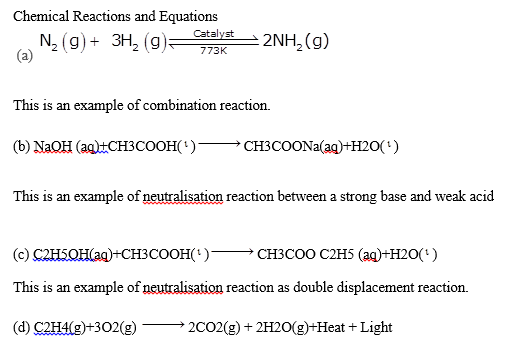This is an example of oxidation reaction.

20. Write the balanced chemical equations for the following reactions and identify the type of reaction in each case.

(a) Thermit reaction, iron (III) oxide reacts with aluminium and gives molten iron and aluminium oxide.

(b) Magnesium ribbon is burnt in an atmosphere of nitrogen gas to form solid magnesium nitride.

(c) Chlorine gas is passed in an aqueous potassium iodide solution to form potassium chloride solution and solid iodine.

(d) Ethanol is burnt in air to form carbon dioxide, water and releases heat.

Soln:

(a) Thermit reaction, iron (III) oxide reacts with aluminium and gives molten iron and aluminium oxide.
FE2O3 + 2Al → Al2O3(s)+ 2Fe (l)

This is a Single displacement reaction

(b) Magnesium ribbon is burnt in an atmosphere of nitrogen gas to form solid magnesium nitride.
3 Mg(s)+ N2 → Mg3N2

The reaction is a Combination reaction

(c) Chlorine gas is passed in an aqueous potassium iodide solution to form potassium chloride solution and solid iodine.
Cl2(g)+2KI (aq) → 2KCl(Aq) +I2(s)

This is a Single displacement reaction

(d) Ethanol is burnt in air to form carbon dioxide, water and releases heat.

C2H5OH+ 3O2 → 2CO2+ 3H2O+ heat

This is a Combustion reaction

21. Complete the missing components/variables given as x and y in the following reactions

(a) Pb(NO3 ) 2 (aq) + 2KI(aq) → PbI2 (x) + 2KNO3 (y)

(b) Cu(s) + 2Ag NO3 (aq) → Cu(NO3 ) 2 (aq) + x(s)

(c) Zn(s) + H2 SO4 (aq) → ZnSO4 (x) + H2 (y)

(d) CaCO3 (s) →x CaO(s) + CO2 (g)

Soln:

(a) Pb(NO3 ) 2 (aq) + 2KI(aq) → PbI2 (s) + 2KNO3 (aq)

(b) Cu(s) + 2Ag NO3 (aq) → Cu(NO3 ) 2 (aq) + 2Ag(s)

(c) Zn(s) + H2 SO4 (aq) → ZnSO4 (aq) + H2 (g)

(d) CaCO3 (s) →heat→ CaO(s) + CO2 (g)

1. x(s), y (aq)
2. x is 2Ag
3. x-(aq) y(g)
4. x is heat

22. Which among the following changes are exothermic or endothermic in nature?

(a) Decomposition of ferrous sulphate

(b) Dilution of sulphuric acid

(c) Dissolution of sodium hydroxide in water

(d) Dissolution of ammonium chloride in water

Soln:

Exothermic reactions

(b) Dilution of sulphuric acid

(c) Dissolution of sodium hydroxide in water

Endothermic reaction

a) Decomposition of ferrous sulphate

(d) Dissolution of ammonium chloride in water

23. Identify the reducing agent in the following reactions

(a) 4NH3 + 5O2 → 4NO + 6H2O

(b) H2O + F2 → HF + HOF

(c) Fe2O3 + 3CO → 2Fe + 3CO2

(d) 2H2 + O2 → 2H2O

Soln:

1. NH3-Ammonia
2. F2- Fluorine
3. CO-Carbon momnoxide
4. H2- Hydrogen

24. Identify the oxidising agent (oxidant) in the following reactions

(a) Pb3O4 + 8HCl → 3PbCl2 + Cl2 + 4H2O

(b) 2Mg + O2 → 2MgO

(c) CuSO4 + Zn → Cu + ZnSO4

(d) V2O5 + 5Ca → 2V + 5CaO

(e) 3Fe + 4H2O → Fe3O4 + 4H2

(f) CuO + H2 → Cu + H2O

Soln:

1. Pb3O4
2. O2
3. CuSO4
4. V2O5
5. H2O
6. CuO

25. Write the balanced chemical equations for the following reactions

(a) Sodium carbonate on reaction with hydrochloric acid in equal molar concentrations gives sodium chloride and sodium hydrogencarbonate.

(b) Sodium hydrogencarbonate on reaction with hydrochloric acid gives sodium chloride, water and liberates carbon dioxide.

(c) Copper sulphate on treatment with potassium iodide precipitates cuprous iodide (Cu2 I2 ), liberates iodine gas and also forms potassium sulphate.

Soln:

(a) Na2CO3 + HCl → NaCl + NaHCO3

(b) NaHCO3 + HCl → NaCl + H2O + CO2

(c) 2CuSO4+4Kl →2K2SO4+CU2I2+I2

26. A solution of potassium chloride when mixed with silver nitrate solution, an insoluble white substance is formed. Write the chemical reaction involved and also mention the type of the chemical reaction?

Soln:

KCl(aq) + AgNO3(aq) → AgCl(s) + KNO3(aq)

This is a double displacement and precipitation reaction.

27. Ferrous sulphate decomposes with the evolution of a gas having a characteristic odour of burning sulphur. Write the chemical reaction involved and identify the type of reaction.

Soln

2FeSO4=Fe2O3+SO2+SO3

This is a decomposition reaction.

28. Why do fire flies glow at night?

Soln:

Fire flies produces an enzyme called as luciferase which carries oxidation of Magnesium Because of oxidation of Magnesium in presence of atmospheric oxygen fireflies glows in the night.

29. Grapes hanging on the plant do not ferment but after being plucked from the plant can be fermented. Under what conditions do these grapes ferment? Is it a chemical or a physical change?

Soln:

Grapes on the plant do not ferment because of defense mechanism of plants. When grapes are plucked from plant grapes reacts with yeast to carry out fermentation. Here sugar changes to alcohol and it is a chemical change.

30. Which among the following are physical or chemical changes?

(a) Evaporation of petrol

(b) Burning of Liquefied Petroleum Gas (LPG)

(c) Heating of an iron rod to red hot.

(d) Curdling of milk

(e) Sublimation of solid ammonium chloride

Soln:

Physical changes

1. Evaporation of petrol
2. Heating of an iron rod to red hot.
3. Sublimation of solid ammonium chloride

Chemical changes

1. Burning of Liquefied Petroleum Gas (LPG)
2. (d) Curdling of milk

31. During the reaction of some metals with dilute hydrochloric acid, following observations were made.

(a) Silver metal does not show any change

(b) The temperature of the reaction mixture rises when aluminium (Al) is added.

(c) The reaction of sodium metal is found to be highly explosive

1. Some bubbles of a gas are seen when lead (Pb) is reacted with the acid.

Explain these observations giving suitable reasons.

Soln:

1. There will not be any reaction between silver and dilute HCL as silver lies in low reactive series of metals.
2. Because it is an exothermic reaction temperature increases
3. Sodium is highly reactive metals it reacts with atmospheric oxygen to form exothermic reaction which result in temperature increase.
4. When lead reacts with acid it produces Hydrogen gas which are responsible for the formation of bubbles.

32. A substance X, which is an oxide of a group 2 element, is used intensively in the cement industry. This element is present in bones also. On treatment with water it forms a solution which turns red litmus blue. Identify X and also write the chemical reactions involved.

Soln:

Compound X is Calcium oxide. CaO is extensively used in cement industry. On treatment with water Cao produces Ca(OH)2 which is alkaline in nature and turns red litmus to blue color.

Ca +H2O→ Ca(OH)2

33. Write a balanced chemical equation for each of the following reactions and also classify them.

(a) Lead acetate solution is treated with dilute hydrochloric acid to form lead chloride and acetic acid solution.

(b) A piece of sodium metal is added to absolute ethanol to form sodium ethoxide and hydrogen gas.

(c) Iron (III) oxide on heating with carbon monoxide gas reacts to form solid iron and liberates carbon dioxide gas.

(d) Hydrogen sulphide gas reacts with oxygen gas to form solid sulphur and liquid water.

Soln:

(a) Pb(CH3COO)2 + 2HCI – PbCl2 + CH3COOH

This is a Double Displacement reaction.

(b) 2Na + 2C2H5OH + 2C2H5ONa+ H2

This is a Displacement reaction.

\(c) Fe2O3 + 3CO + 2Fe + 3CO2

This is a redox reaction.

(d) 2 H2S+O→ 2 s + 2 H2O

This is a replacement reaction.

34. Why do we store silver chloride in dark coloured bottles?

Soln:

Silver chloride decomposes into silver and chlorine gas when exposed to sunlight. Hence Silver chloride is stored in dark colored bottles.

35. Balance the following chemical equations and identify the type of chemical reaction.

(a) Mg(s) + Cl2 (g) → MgCl2 (s)

(b) HgO(s) → Heat Hg(l) + O2 (g)

(c) Na(s) + S(s) → Fuse Na2S(s)

(d) TiCl4 (l) + Mg(s) → Ti(s) + MgCl2 (s)

(e) CaO(s) + SiO2 (s) → CaSiO3 (s)

(f) H2O2 (l) → U V H2O(l) + O2 (g)

Soln:

(a) Mg(s) + Cl2(g) → MgCl2(s)

This type of reaction is called a combination reaction or a synthesis reaction.

(b) 2HgOis) — (Heat) → 2 Hg(I) + O2(g)

This is an example of thermal decomposition reaction.

(c) 2Na(s) + S(s) — (Fuse) → Na2S(s)

This is an example of a Combination reaction.

(d) TiCI4 (1) + Mg(s) → Ti(s) + 2MgCl2 (s)

This reaction falls under the category of Displacement reactions

(e) Cao(s) + SIO2(s) + CaSIO3(s)

This is a synthesis reaction.

(f) 2H2O2 (I) — UV → 2H2O(I) + O2 (g)

This is a decomposition reaction.

36. A magnesium ribbon is burnt in oxygen to give a white compound X accompanied by emission of light. If the burning ribbon is now placed in an atmosphere of nitrogen, it continues to burn and forms a compound Y.

(a) Write the chemical formulae of X and Y.

(b) Write a balanced chemical equation, when X is dissolved in water.

Soln:

2Mg + O₂ →2MgO

Compound X→ MgO  [ Magnesium Oxide ]

Compound Y →Mg₃N₂  [ Magnesium Nitride ]

If Magnesium Oxide is dissolved in water , the product is Magnesium Hydroxide

MgO  + H₂O → Mg(OH)₂

37. Zinc liberates hydrogen gas when reacted with dilute hydrochloric acid, whereas copper does not. Explain why?

Soln:

Zinc is more reactive than copper as Zinc is placed above Hydrogen and Copper is placed below hydrogen in the activity series of metals. Because of this Zinc reacts with HCl whereas Copper will not react.

38. A silver article generally turns black when kept in the open for a few days. The article when rubbed with toothpaste again starts shining. (a) Why do silver articles turn black when kept in the open for a few days? Name the phenomenon involved. (b) Name the black substance formed and give its chemical formula.

Soln:

1. Silver reacts with H2S present in the atmosphere to form a black color compound Silver Sulphide. This phenomenon is called as corrosion.
2. Black color compound formed is Silver Sulphide

2Ag+ H2S →Ag2S+H2

39. On heating blue coloured powder of copper (II) nitrate in a boiling tube, copper oxide (black), oxygen gas and a brown gas X is formed

(a) Write a balanced chemical equation of the reaction.

(b) Identity the brown gas X evolved.

(c) Identity the type of reaction.

(d) What could be the pH range of aqueous solution of the gas X?

Soln:

(a)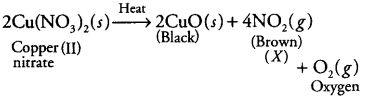(b) The brown gas X is nitrogen dioxide, NO2.

(c) Reaction involved is Thermal decomposition.

(d) pH< 7 because NO2 dissolves in water to form acidic solution (pH range below 7).

40. Give the characteristic tests for the following gases

(a) CO2

(b) SO2

(c) O2

(d) H2

Soln:

1. Pass CO2 into limewater which will turn water into milky. This is the confirmation test for the presence of Carbon-di-oxide.
2. Smell is the characteristic feature of SO2 which smells like rotten egg.
3. Test for oxygen involves burning of match stick near oxygen makes it burn even more brightly.
4. When Burning matchstick is brought near H2 gas, the flame burns with the pop sound. This is the test to confirm Hydrogen gas.

41. What happens when a piece of

(a) zinc metal is added to copper sulphate solution?

(b) aluminium metal is added to dilute hydrochloric acid?

(c) silver metal is added to copper sulphate solution?

Also, write the balanced chemical equation if the reaction occurs

Soln:

a) When Zinc is added to copper sulphate solution Zinc displaces copper to form Zinc sulphate.

Zn(s)+ CuSo4(aq)→ ZnSo4(aq)+ Cu(s)

b)Alluminium metals reacts with dilute HCl to for Aluminum Chloride and Hydrogen gas is evolved in the reaction.

2Al (s)+ 6HCl(aq)→ 2AlCl3(aq)+ 3 H2

c)When silver metal is added to Copper Sulphate solution there will not be any reaction as silver is non-reactive metal.

42. What happens when zinc granules are treated with dilute solution of H2SO4 , HCl, HNO3 , NaCl and NaOH, also write the chemical equations if reaction occurs.

Soln:

When Zinc granules reacts with dil H2SO4 displacement reaction takes place leading to the formation of ZnSo4 liberating H2 gas

Zn( s)+ H2SO4(aq)→ ZnSo4 (aq)+H2 (g)

When Zinc granules reacts with dil HCl displacement reaction takes place leading to the formation of ZnCl2 liberating H2 gas

Zn( s)+ H2Cl(aq) →ZnCl2 (aq)+H2 (g)

When Zinc granules reacts with dil HNO3 it leads to the formation of Zinc nitrate evolving H2O and nitrous oxide

4Zn(s)+10 HNO3(aq) 4Zn(NO3)2(aq) +5H2O(i)+N2O (g)

When Zinc granules reacts with Nacl there will not be any reaction

When Zinc granules reacts with NaOH solution

Zn(s)+ NaOH(aq)→ Na2ZnO2 (aq)+H2 (g)

43. On adding a drop of barium chloride solution to an aqueous solution of sodium sulphite, white precipitate is obtained.

(a) Write a balanced chemical equation of the reaction involved

(b) What other name can be given to this precipitation reaction?

(c) On adding dilute hydrochloric acid to the reaction mixture, white precipitate disappears. Why?

Soln:

a)On adding a drop of Barium Chloride solution to an aqueous solution of sodium sulphite, barium sulphite is obtained which is white colour precipitate.

BaCl2 + Na2SO3——>BaSO3 + 2NaCl

b) In this case precipitation reaction is a double displacement.

c) When we add dilute HCl to this reaction mixture Barium chloride, Sulphur dioxide and water are formed. Barium chloride is a soluble substance which will make the white precipitate disappear.

BaSO3 + HCl→ BaCl3 + SO2 + H2O.

44. You are provided with two containers made up of copper and aluminium. You are also provided with solutions of dilute HCl, dilute HNO3 , ZnCl2 and H2O. In which of the above containers these solutions can be kept?

These solution can be kept in copper container because copper is a noble metal which will not react with HCl or even HNO3. If we keep solution in Aluminum container aluminum reacts with acids to for Zinc chloride.

Water can be stored in either copper or aluminum as they both aluminum and copper are very less reactive with copper.

## NCERT Exemplar Class 10 Science Chemical Reactions

This solution has answers to the questions given in the NCERT Exemplars together with chemical reactions and equations class 10 questions answers, MCQ’S, fill in the blanks, match the following kind of questions.

Chemical reactions are very fundamentals topic which will help you in all your future studies. Hence students are advised to get the knowledge of this chapter thoroughly

## Important topics of Class 10 Chapter 1 Science Chemical Reactions and Equations

 Section Number Topic 1.1 Chemical Equations 1.1.1 Writing A Chemical Equation 1.1.2 Balanced Chemical Equations 1.2 Types Of Chemical Reactions 1.2.1 Combination Reaction 1.2.2 Decomposition Reaction 1.2.3 Displacement Reaction 1.2.4 Double Displacement Reaction 1.3 Have You Observed The Effects Of Oxidation Reactions In Everyday Life? 1.3.1 Corrosion 1.3.2 Rancidity

In This Chapter students will get the basic knowledge about a chemical reaction, writing and balancing chemical equations, types of chemical reaction, effects of oxidation reactions, explanation about chemical reaction behind corrosion and rancidity.

Students are also advised to solve the CBSE previous year question as well as the sample papers. Solving the sample papers and CBSE previous year papers will also help them to know the exam pattern as well as the marking scheme.

## Frequently Asked Questions on chemical reactions and equation

### Why Zinc liberates hydrogen gas when reacted with dilute hydrochloric acid, whereas copper does not ?

Zinc is more reactive than copper as Zinc is placed above Hydrogen and Copper is placed below hydrogen in the activity series of metals. Because of this Zinc reacts with HCl whereas Copper will not react.

### Why do we store silver chloride in dark coloured bottles ?

Silver chloride decomposes into silver and chlorine gas when exposed to sunlight. Hence Silver chloride is stored in dark colored bottles.

### Why do fire flies glow at night ?

Fire flies produces an enzyme called as luciferase which carries oxidation of Magnesium Because of oxidation of Magnesium in presence of atmospheric oxygen fireflies glows in the night.